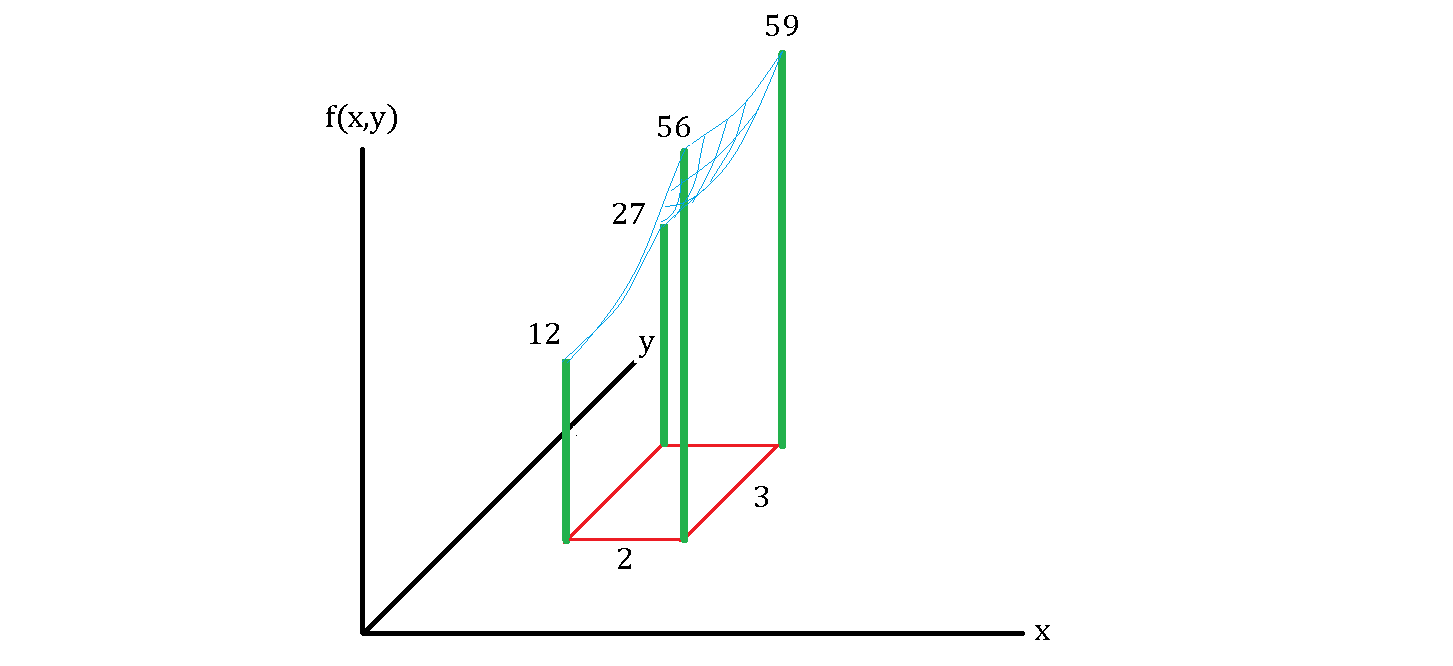# Bamboo Forest

You are surveying a rectangular area of a bamboo forest of $2 \times 3$ square feet. The four bamboos at the corners are each $12, 27, 56, 59$ feet high, and when you analyze the surface area at the top, you find that it is a partial plain of $f(x,y) = y^2 + x^3 - 2xy +7,$ as shown below.

Assuming the area is densely packed with bamboos, what is the average height of these bamboos in feet?×